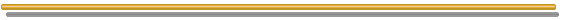# FAQ Number 5

## Is Possible to Use Real Options for Incomplete Markets? What Changes? What Are the Possible Ways?

Answer: Yes, is possible to use.

• For incomplete markets the risk-neutral probability (martingale measure) is not unique;
• So, risk-neutral valuation is not rigourously correct here because there is a lack of market values;
• Academicians and practitioners use some ways to estimate the real option value with incomplete markets.

In case of incomplete market, the alternatives for real options valuation are:

1. Assume that the market is approximately complete (your estimative of market value is reliable) and use risk-neutral valuation (with risk-neutral probability);
2. Assume firms are risk-neutral and discount with risk-free interest rate (with real probability);
3. Specify preferences (the utility function) of single-agent or the equilibrium at detailed level (see Duffie's textbook, 1996).
This is used by finance academicians.
In practice is difficult, if not impossible, to specify the utility of a corporation (managers, stockholders);
4. Use the dynamic programming framework with an exogenous discount rate
Used by economists from academy: Dixit & Pindyck, Lucas, etc.
Corporate discount rate express the corporate preferences? This is difficult to answer but most of time the discount rate is the only observable referential risk-preference in a corporation.Go to the Next FAQ: 6) Is true that mean-reversion always reduces the options premium? What is the effect of jumps in the option premium?

Back to the FAQ's ListBack to Contents